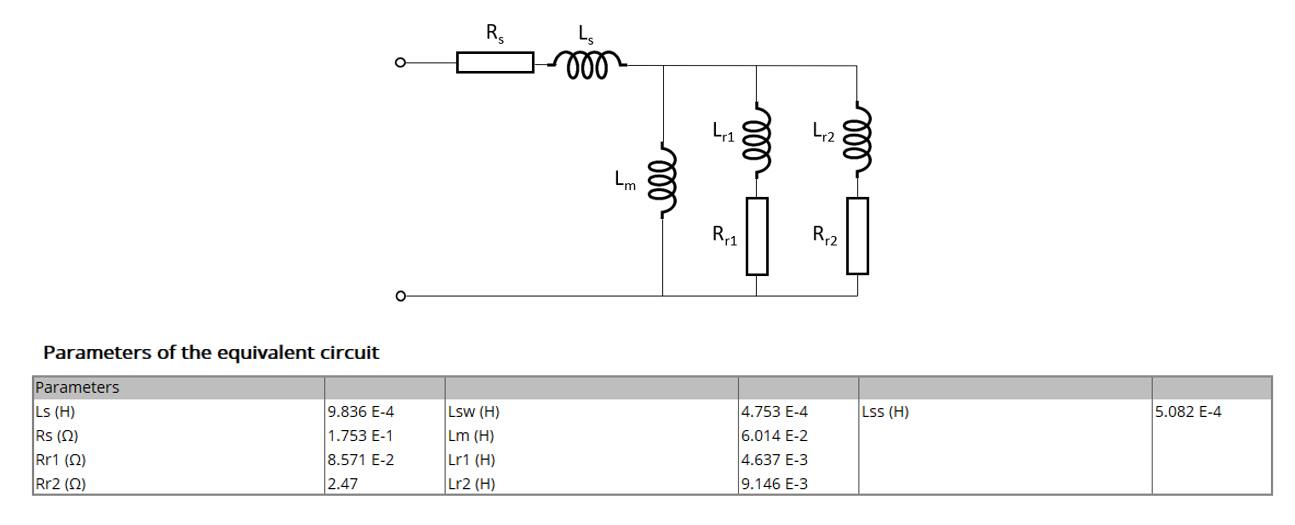# Char. - Model - Motor & Generator - SSFR

## 1. Positioning and objective

The aim of the test “ Characterization – Model – Motor – SSFR ” is to characterize all the parameters of the equivalent scheme by performing a frequency analysis.

Then, the resulting equivalent scheme can be evaluated by simulating the starting of the considered induction machine. The speed, torque and absorbed current versus time are computed and displayed.“Characterization – Model – Motor – SSFR” – Characterization of all the equivalent scheme parameters

These results are based on the magnitude and the phase of the operational inductance transfer function which are computed with Finite Element software.

The following table helps to classify the test “Characterization – Model – Motor – SSFR”.

 Family Characterization Package Model Convention Motor Test SSFR Positioning of the test “Characterization – Model – Motor – SSFR”

## 2. User inputs

The main user input parameter is the order of the operational inductance transfer function. 1 st and 2 nd order are considered depending on the squirrel cage topology but also on the results accuracy got with the 1 st order model.

Then, the Line-Line voltage and the power supply frequency must be given to compute instantaneously, the induction motor starting based on the resulting equivalent scheme.

In addition, temperatures of stator winding and squirrel cage must be set.

## 3. Main outputs

The main outputs are all the computed parameters of the equivalent scheme (First or second order).

The quality of results is also illustrated with the superimposition of the magnitude and phase of the operational inductance is computed either with Finite Element software Flux2D (Steady State AC application) or analytically by considering the resulting operational inductance.

## 3.1 Table or results

1. Equivalent scheme parameters

• Operational inductance Laplace function with the corresponding computed parameters
• Induction machine equivalent scheme (first and second order) with its associated computed parameters
• Model evaluation – Start-up characteristics

## 3.2 Curves

1. Magnitude of the operational inductance versus frequency
2. Phase of the operational inductance versus frequency
3. Model evaluation – Speed versus time
4. Model evaluation – Torque versus time
5. Model evaluation – Current versus time